# liquidSVM: Demo for R

#### 2017-07-15

We give a demonstration of the capabilities of liquidSVM from an R viewpoint. More detailed information can be found in the documentation and in the help (e.g. ?svm).

Disclaimer: liquidSVM and the R-bindings are in general quite stable and well tested by several people. However, use in production is at your own risk.

If you run into problems please check first the documentation for more details, or report the bug to the maintainer.

## liquidSVM in one Minute

To install and load the library, start an R session and type

install.packages("liquidSVM")
library(liquidSVM)

LS-Regression:

# Load test and training data
reg <- liquidData('reg-1d')

Now reg$train contains the training data and reg$test the testing data. Both have the labels in its first column Y and the feature is in column X1. To train on the data and select the best hyperparameters do (display=1 gives some information as the training progresses)

model <- svm(Y~., reg$train) #> Warning in rm(model): Objekt 'model' nicht gefunden Now you can test with any test set: result <- test(model, reg$test)
errors(result)
#> 0.00541

We also can plot the regression:

plot(reg$train$X1, reg$train$Y,pch='.', ylim=c(-.2,.8), ylab='', xlab='', axes=F)
curve(predict(model, x),add=T,col='red')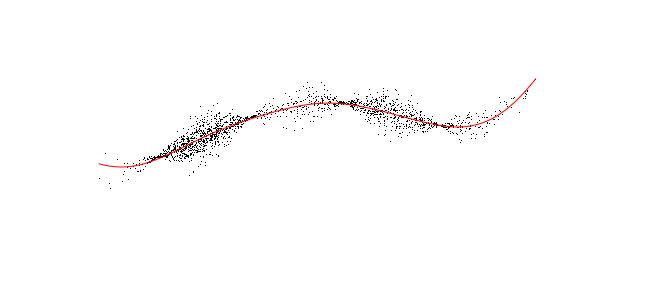As a convenience, since reg already contains $train and $test you can do the whole experiment in one line. Then the result is stored in model$last_result: model <- svm(Y~., reg, display=1) errors(model$last_result)
#> 0.00541

Multi-class:

banana <- liquidData('banana-mc')
banana
#> LiquidData "banana-mc" with 4000 train samples and 4000 test samples
#>   having 3 columns named Y,X1,X2
#>   target "Y" factor with 4 levels: 1 (1200 samples) 2 (1200 samples) 3 (800 samples) ...

Since banana$train$Y is a factor the following performs multi-class classification

model <- svm(Y~., banana$train) plot(banana$train$X1, banana$train$X2,pch='o', col=banana$train$Y, ylab='', xlab='', axes=F) x <- seq(-1,1,.05) z <- matrix(predict(model,expand.grid(x,x)),length(x)) contour(x,x,z, add=T, levels=1:4,col=1,lwd=4)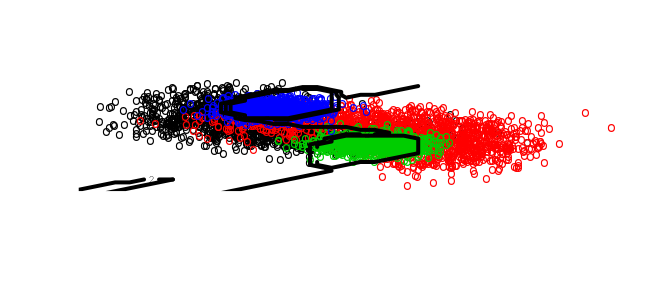In this case errors(...) shows both the global miss-classification error as well the errors of the underlying binary tasks, for more details see Multiclass classification: errors(test(model,banana$test))
#>   result     1vs2     1vs3     1vs4     2vs3     2vs4     3vs4
#> 0.218000 0.141250 0.112500 0.095500 0.075500 0.075000 0.000625

Cells: if data gets too big for the memory on your machine:

covtype <- liquidData('covtype.5000')
model <- svm(Y~., covtype, display=1, useCells=TRUE)
errors(model$last_result) #> 0.192 ## First steps Download and installation can be done from a running R session by install.packages("liquidSVM") If you have CUDA installed e.g. on path /usr/local/cuda you can activate GPU support at installation by install.packages('liquidSVM',configure.args="native /usr/local/cuda") At the moment this is not tested on Windows! Once the package is installed you can open the very vignette you are reading by issuing: vignette('demo',package='liquidSVM') After Installation you have to load the package library(liquidSVM) To get started we consider two problems using R-builtin datasets: • Regression with the trees data set (31 samples, 3 variables) and we use the formula Height ~ Girth + Volume This means that Height of trees should be explained by both their Girth and Volume. • Multi-classification with the iris data set (150 samples, 5 variables): Species ~ . The factor Species has 3 levels setosa, versicolor, and virginica. Since we are explaining a factor this yields by default a multi-classification problem. Note that the dot on the right hand side means that all the other variables of iris are to be used to explain. Hence this is equivalent to: Species ~ Sepal.Length + Sepal.Width + Petal.Length + Petal.Width For the most simple out of the box training use svm(...). If nothing else is specified then the explanatory variable determines what kind of problem is getting addressed: modelTrees <- svm(Height ~ Girth + Volume, trees) # least squares modelIris <- svm(Species ~ ., iris) # multiclass classification #> Warning in (function (model, command.args = NULL, ..., d = NULL, #> warn.suboptimal = getOption("liquidSVM.warn.suboptimal", : Solution may not #> be optimal: try training again using max_gamma=25 This trains a model and can take some time for bigger data sets. It issues 5-fold cross-validation on a grid of 10 bandwidth parameters gamma and 10 cost parameters lambda. Also the best parameters are selected by default. Hence after this one can calculate predictions for the model as usual in R: predict(modelTrees, trees[21:31, ]) #>  78.8 78.4 74.9 73.3 76.5 81.5 81.5 80.3 80.0 79.8 84.7 predict(modelIris, iris[3:8, ]) #>  setosa setosa setosa setosa setosa setosa #> Levels: setosa versicolor virginica The actual values that liquidSVM produces can vary on several accounts: There are random components in the solving process and also different architectures yield different optimization strategies and hence different near-to-optimal solutions. This holds for all the outputs in this document. The training on the full iris data gives a perfect fit: all.equal( predict(modelIris, iris[3:8, ]) , iris$Species[3:8] )
#>  TRUE

The better approach to machine learning is to split the samples into a training set and a test set. Here we split earthquakes on fiji (1000 seismic events, 5 numerical values) in a training set of size

qu <- ttsplit(quakes)

Then we learn on the training set:

model <- svm(mag ~ ., qu$train) and test on the testing set: result <- test(model, qu$test)
errors(result)
#> val_error
#>    0.0336

Since qu already has both a training and a testing data set, we can do train/select and test in one invocation (and give more information using display=1)

model <- lsSVM(mag ~ . , qu, display=1)
#> [...]
#> Warning: The best gamma was 0 times at the lower boundary and 5 times at the
#> upper boundary of your gamma grid. 5 times a gamma value was selected.
#> [...]
errors(model$last_result) #> val_error #> 0.109 This error feels to big, but there is some information that the gamma-grid is to small. Hence we use bigger and bigger grids: errors(lsSVM(mag ~ . , qu, max_gamma=100)$last_result)
#> val_error
#>    0.0367

and this is much better.

Solutions can be saved as in the following examples (taken from the examples of read.liquidSVM):

banana <- liquidData('banana-bc')
modelOrig <- mcSVM(Y~., banana$train) write.liquidSVM(modelOrig, "banana-bc.fsol") write.liquidSVM(modelOrig, "banana-bc.sol") clean(modelOrig) # delete the SVM object # now we read it back from the file modelRead <- read.liquidSVM("banana-bc.fsol") # No need to train/select the data! errors(test(modelRead, banana$test))

# to read the model where no data was saved we have to make sure, we get the same training data:
banana <- liquidData('banana-bc')
# then we can read it
modelDataExternal <- read.liquidSVM("banana-bc.sol", Y~., banana$train) result <- test(modelDataExternal, banana$test)

# to serialize an object use:
banana <- liquidData('banana-bc')
modelOrig <- mcSVM(Y~., banana$train) # we serialize it into a raw vector obj <- serialize.liquidSVM(modelOrig) clean(modelOrig) # delete the SVM object # now we unserialize it from that raw vector modelUnserialized <- unserialize.liquidSVM(obj) errors(test(modelUnserialized, banana$test))

### Cells

A major issue with SVMs is that for larger sample sizes the kernel matrix does not fit into the memory any more. Classically this gives an upper limit for the class of problems that traditional SVMs can handle without significant runtime increase. The concept of cells makes it possible to circumvent these issues.

If you specify useCells=TRUE then the sample space $$X$$ gets partitioned into a number of cells. The training is done first for cell 1 then for cell 2 and so on. Now, to predict the label for a value $$x\in X$$ liquidSVM first finds out to which cell this $$x$$ belongs and then uses the SVM of that cell to predict a label for it:

banana <- liquidData('banana-bc')
model <- mcSVM(Y~.,banana$train, voronoi=c(4,500)) centers <- getCover(model)$samples
plot(banana$train[,2:3],col=banana$train$Y) points(centers,pch='x',cex=2,col=3) if(require(deldir)){ voronoi <- deldir::deldir(centers$X1,centers$X2,rw=c(range(banana$train$X1),range(banana$train$X2))) plot(voronoi,wlines="tess",add=TRUE, lty=1) text(centers$X1,centers$X2,1:nrow(centers),pos=1) }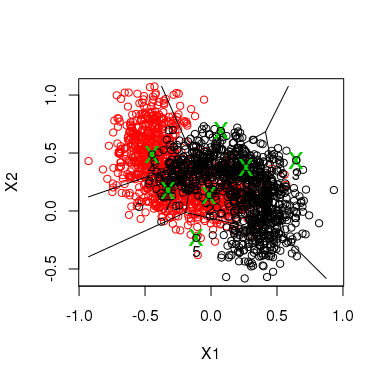We first consider a medium size sample of the covtype data set. liquidData will download this from http://www.isa.uni-stuttgart.de/liquidData/: co <- liquidData('covtype.10000') system.time(svm(Y~., co$train, threads=3))
#>   user  system elapsed
#> 28.208   0.124  11.191 

The user time is about three times the elapsed time since we are using 3 threads.

By using the partitioning facility of liquidSVM you can even bigger problems:

co <- liquidData('covtype.50000')
system.time(svm(Y~.,co$train,useCells=TRUE,threads=3)) #> user system elapsed #> 252.395 1.076 98.119 Note that with this data set useCells=F here only works if your system has enough free memory (~26GB). Even the full covtype data set with over 460’000 rows (about 110’000 samples retained for testing) is now treatable in under 7 minutes from within R: co <- liquidData('covtype-full') system.time(svm(Y~.,co$train,useCells=TRUE,threads=3))
#>     user   system  elapsed
#> 1383.535    4.752  397.559

If you have less than 10GB of RAM use store_solutions_internally=FALSE for the latter.

If you run into memory issues turn cells on: useCells=TRUE

### CUDA

liquidSVM also is able to calculate the kernel on a GPU if it is compiled with CUDA-support. Since there is a big overhead in moving the kernel matrix from the GPU memory, this is most useful for problems with many dimensions. We take here the Gisette data set which takes the digits 4 and 9 from the standard MNIST data of handwritten digits and adds some new attributes to obtain 6000 samples and 5000 attributes.

First we load the data into a liquidSVM-model:

gi <- liquidData('gisette')
model <- init.liquidSVM(Y~.,gi$train) Now we train the model with and without GPU to compare: system.time(trainSVMs(model,d=1,gpus=1,threads=1)) #> user system elapsed #> 57 10 67 system.time(trainSVMs(model,d=1,gpus=0,threads=4)) #> user system elapsed #> 392 1 110 Note that liquidSVM uses only as much threads as GPUs if GPUs are used at all, hence there is no elapsed time gain in comparison to 4 threads: system.time(trainSVMs(model,d=1,gpus=1,threads=4)) #> user system elapsed #> 94 42 67 system.time(trainSVMs(model,d=1,gpus=0,threads=1)) #> user system elapsed #> 327 1 329 ### Comparison to libsvm We use e1071::svm, which is a binding for libsvm. You can install it from CRAN by using install.packages("e1071") liquidSVM’s out of the box behavior is to use cross-validation: folds <- 5 co <- liquidData('covtype.1000') system.time(ours <- svm(Y~., co$train, folds=folds, threads=2))
#>   user  system elapsed
#>  1.525   0.016   0.958

The user-time is about twice the elapsed since we use 2 threads here.

How can the same be achieved in e1071::svm? First the parameter-search grid has to be converted:

GAMMA <- 1/(ours$gammas)^2 COST <- 1/(2 * (folds-1)/folds * nrow(co$train) * ours$lambdas) And then we use e1071::tune.svm to perform the cross-validation system.time(e1071::tune.svm(Y~., data=co$train, gamma=GAMMA,cost=COST, scale=F, e1071::tune.control(cross=folds)))
#>   user  system elapsed
#> 382.364   0.832 385.521  

Now, for bigger datasets this gives a consistent picture:

co <- liquidData('covtype.5000')  # ca. 5000 rows
system.time(ours <- svm(Y~., co$train, folds=folds, threads=2)) #> user system elapsed #> 30.237 0.120 15.676 system.time(e1071::tune.svm(Y~., data=co$train, gamma=GAMMA,cost=COST, scale=F, e1071::tune.control(cross=folds)))
#>      user    system   elapsed
#> 11199.732     4.324 11238.407

liquidSVM is better also if training is done only on one grid:

co <- liquidData('covtype.10000')  # ca. 10000 rows
gamma <- 3.1114822
cost <- 0.01654752
system.time(ours <- svm(Y ~ ., co$train, g=c("[",gamma,"]"), l=c("[",cost,"]",1),folds=1,threads=4,d=1)) #> user system elapsed #> 4.836 0.356 2.134 system.time(theirs <- e1071::svm(Y~., co$train, gamma=1/gamma^2,cost=cost, scale=F))
#>   user    system   elapsed
#> 26.502     0.032    26.618 

This 10-times speed-up is possible on one hand due to more efficient computations of the kernel matrix and on the other hand due to liquidSVM’s faster optimization algorithm.

Again for bigger problems this holds true:

co <- liquidData('covtype.35000')  # ca. 35000 rows
system.time(ours <- svm(Y ~ ., co$train, g=c("[",gamma,"]"), l=c("[",cost,"]",1),folds=1,threads=4,d=1)) #> user system elapsed #> 99.830 4.544 36.949 system.time(theirs <- e1071::svm(Y~., co$train, gamma=1/gamma^2,cost=cost, scale=F))
#>    user  system elapsed
#> 330.557   0.176 331.834 

To compare to the same big data set using full 10x10 grid search but just with one fold is still quicker:

system.time(ours <- svm(Y ~ ., co$train,folds=1,threads=4,d=0)) #> user system elapsed #> 816.475 5.164 225.934  Basically in the time libsvm does one solution you get the whole grid search for free if you use liquidSVM. ## Learning Scenarios liquidSVM organizes its work into tasks: E.g. in multiclass classification the problem has to be reduced into several binary classification problems. Or in Quantile regression, the SVM is learned simultaneously for different weights and then the selection of hyperparameters produces different tasks. Behind the scenes svm(formula, data, ...) does the following: model <- init.liquidSVM(formula, data) trainSVMs(model, ...) selectSVMs(model) The following learning scenarios hide these in higher level functions. ### Multiclass classification Multiclass classification has to be reduced to binary classification There are two strategies for this: • all-vs-all: for every pairing of classes a binary SVM is trained • one-vs-all: for every class a binary SVM is trained with that class as one label and all other classes are clumped together to another label Then for any point in the test set, the winning label is chosen. A second choice to make is whether the hinge or the least-square loss should be used for the binary classification problems. Let us look at the example dataset banana-mc which has 4 labels: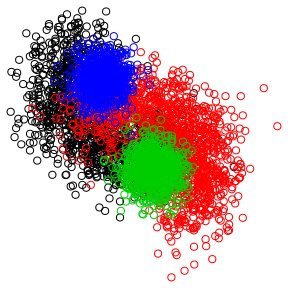Since there are 6 pairings, AvA trains 6 tasks, whereas OvA trains 4 tasks: banana <- liquidData('banana-mc') model <- mcSVM(Y~., banana, mc_type="AvA_hinge") errors(model$last_result)
#>   result     1vs2     1vs3     1vs4     2vs3     2vs4     3vs4
#> 0.217250 0.142083 0.111500 0.092500 0.073500 0.073500 0.000625
model$last_result[1:3,] #> result 1vs2 1vs3 1vs4 2vs3 2vs4 3vs4 #> 1 1 1 1 1 2 4 4 #> 2 4 1 1 4 2 4 4 #> 3 4 1 1 4 2 4 4 model <- mcSVM(Y~., banana, mc_type="OvA_ls") errors(model$last_result)
#>    result 1vsOthers 2vsOthers 3vsOthers 4vsOthers
#>    0.2147    0.1545    0.1227    0.0777    0.0737
model$last_result[1:3,] #> result 1vsOthers 2vsOthers 3vsOthers 4vsOthers #> 1 1 0.99149 -0.964 -0.924 -0.928 #> 2 4 -0.45494 -1.000 -0.994 0.387 #> 3 1 -0.00657 -0.991 -0.993 -0.111 model <- mcSVM(Y~., banana, mc_type="AvA_ls") errors(model$last_result)
#>   result     1vs2     1vs3     1vs4     2vs3     2vs4     3vs4
#> 0.212500 0.140000 0.107000 0.089500 0.074000 0.074000 0.000625
model$last_result[1:3,] #> result 1vs2 1vs3 1vs4 2vs3 2vs4 3vs4 #> 1 1 -0.963 -0.979 -0.9966 -0.605 0.894 0.995 #> 2 4 -0.753 -0.998 0.5268 -0.953 1.000 1.000 #> 3 1 -0.996 -1.000 -0.0506 -0.894 1.000 1.000 # For completeness the following is also possible even though you should not use it: model <- mcSVM(Y~., banana, mc_type="OvA_hinge") errors(model$last_result)
#>    result 1vsOthers 2vsOthers 3vsOthers 4vsOthers
#>    0.2235    0.1555    0.1275    0.0795    0.0750
model$last_result[1:3,] #> result 1vsOthers 2vsOthers 3vsOthers 4vsOthers #> 1 1 1.000 -0.829 -0.720 -0.981 #> 2 4 -0.876 -0.995 -0.740 0.923 #> 3 4 -0.202 -0.987 -0.729 0.198 The first element in the errors gives the overall test error. The other errors correspond to the tasks. Also the result displays in the first column the final decision for a test sample, and in the other columns the results of the binary classifications. One can see nicely how the final prediction vote for any sample is based on the 4 or 6 binary tasks. NOTE AvA is usually faster, since every binary SVM just trains on the data belonging to only two labels. On the other hand OvA_ls can give better results at the cost of longer training time. OvA_hinge should not be used as it is not universally consistent. ### Quantile regression This uses the quantile solver with pinball loss and performs selection for every quantile provided. reg <- liquidData('reg-1d') quantiles_list <- c(0.05, 0.1, 0.5, 0.9, 0.95) model <- qtSVM(Y ~ ., reg$train, weights=quantiles_list)

result_qt <- test(model,reg$test) errors(result_qt) #>  0.00714 0.01192 0.02682 0.01251 0.00734 Now we plot this: I <- order(reg$test$X1) par(mar=rep(.1,4)) plot(Y~X1, reg$test[I,],pch='.', ylim=c(-.2,.8), ylab='', xlab='', axes=F)
for(i in 1:length(quantiles_list))
lines(reg$test$X1[I], result_qt[I,i], col=i+1)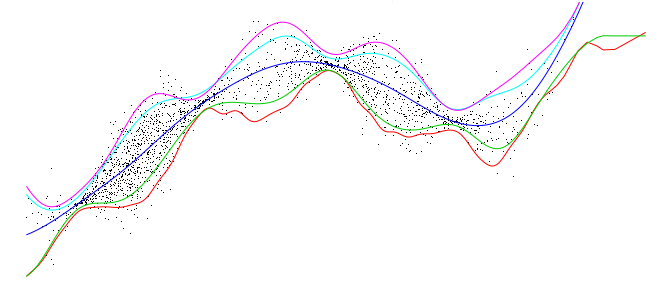In this plot you see estimations for two lower and upper quantiles as well as the median of the distribution of the label $$y$$ given $$x$$.

### Expectile regression

This uses the expectile solver with weighted least squares loss and performs selection for every weight. The 0.5-expectile in fact is just the ordinary least squares regression and hence estimates the mean of $$y$$ given $$x$$. And in the same way as quantiles generalize the median, expectiles generalize the mean.

reg <- liquidData('reg-1d')
expectiles_list <- c(0.05, 0.1, 0.5, 0.9, 0.95)

model <- exSVM(Y ~ ., reg$train, weights=expectiles_list) result_ex <- test(model, reg$test)
errors(result_ex)

Now we plot this:

I <- order(reg$test$X1)
par(mar=rep(.1,4))
plot(Y~X1, reg$test[I,],pch='.', ylim=c(-.2,.8), ylab='', xlab='', axes=F) for(i in 1:length(expectiles_list)) lines(reg$test$X1[I], result_ex[I,i], col=i+1) legend('bottomright', col=6:2, lwd=1, legend=expectiles_list[5:1])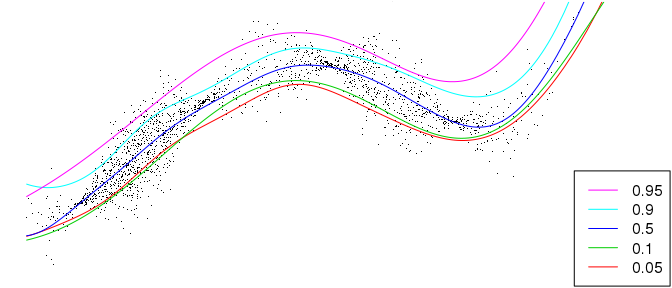### Neyman-Pearson-Learning Neyman-Pearson-Learning attempts classification under the constraint that the probability of false positives (Type-I error) is bound by a significance level alpha, which is called here the NPL-constraint. banana <- liquidData('banana-bc') npl_constraints <- c(0.025,0.033,0.05,0.075,0.1) # class=-1 specifies the normal class model <- nplSVM(Y ~ ., banana, class=-1, constraint.factor=npl_constraints,threads=0,display=1) result_npl <- model$last_result
errors(result_npl)
#>  0.437 0.437 0.322 0.308 0.230

Now how did we do?

false_alarm_rate <- apply(result_npl[banana$test$Y==-1,]==1,2,mean)
detection_rate <- apply(result_npl[banana$test$Y==1,]==1,2,mean)
rbind(npl_constraints,false_alarm_rate,detection_rate)
#>                      1      2     3     4     5
#> npl_constraints  0.025 0.0333 0.050 0.075 0.100
#> false_alarm_rate 0.037 0.0390 0.053 0.058 0.086
#> detection_rate   0.563 0.5630 0.678 0.692 0.770

You can see that the false alarm rate in the test set meet the NPL-constraints quite nicely, and on the other hand the the detection rate is increasing.

### ROC curve

Receiver Operating Characteristic curve (ROC curve) plots trade-off between the false alarm rate and the detection rate for different weights (default is 9 weigts).

banana <- liquidData('banana-bc')

model <- rocSVM(Y ~ ., banana$train, threads=0,display=1) result_roc <- test(model, banana$test)

Now you could quickly plot this curve using plotROC(model, banana$test) but let’s do it by hand: false_positive_rate <- apply(result_roc[banana$test$Y==-1,]==1,2,mean) detection_rate <- apply(result_roc[banana$test$Y==1,]==1,2,mean) plot(false_positive_rate, detection_rate, xlim=0:1,ylim=0:1,asp=1, type='b', pch='x') abline(0,1,lty=2)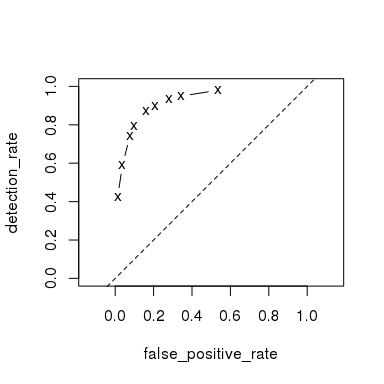This shows nice learning, since the ROC curve is near the north-west corner. A quicker way to calculate an ROC curve is to use least-squares regression which estimates the conditional probability at any point. For this we use the same method plotROC: model.ls <- lsSVM(Y~.,banana$train)
#> Warning in rm(model.ls): Objekt 'model.ls' nicht gefunden
plotROC(model.ls, banana$test, xlim=0:1,ylim=0:1,asp=1, type='l') points(false_positive_rate, detection_rate, pch='x', col='red')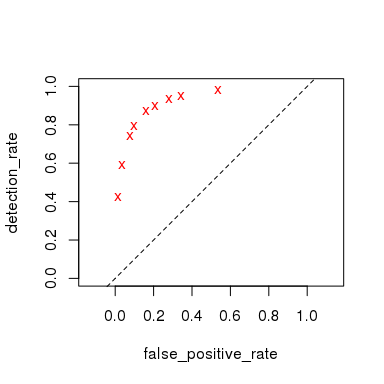And we see that both methods give almost the same results. # Calculating the Kernel Matrix We provide an interface to calculating the kernel matrix of features: covtype <- liquidData("covtype.1000")$train[1:100,-1]
a <- kern(covtype)
a[1:4,1:4]
#>        [,1]   [,2]    [,3]    [,4]
#> [1,] 1.0000 0.0159 0.10593 0.01112
#> [2,] 0.0159 1.0000 0.01296 0.06971
#> [3,] 0.1059 0.0130 1.00000 0.00755
#> [4,] 0.0111 0.0697 0.00755 1.00000
image(liquidSVM::kern(covtype, gamma=1.1, type="gauss"))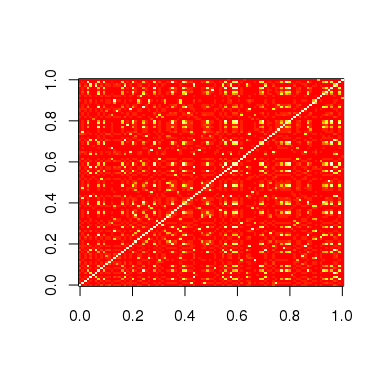image(liquidSVM::kern(covtype, gamma=1.1, type="poisson"))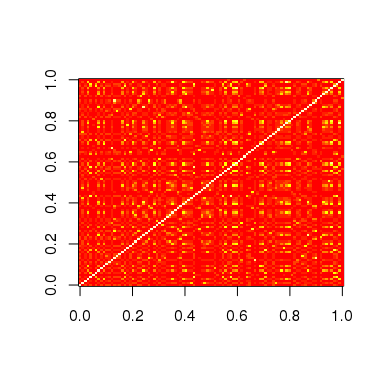# liquidData

As a convenience we provide several datasets prepared for training and testing.

http://www.isa.uni-stuttgart.de/liquidData

They can be imported by name e.g. using:

liquidData('reg-1d')

This loads both reg-1d.train.csv as well as reg-1d.test.csv into reg$train and reg$test respectively.

liquidData sets have a strict format, they are comma-separated values and no header. The first column is the label. It gets the variable name Y. The other columns are the features and get variable names X1, X2,

You can also load samples as in the following examples

# take 10% of training and testing data
liquidData('reg-1d', prob=0.1)
# a sample of 400 train samples and the same relative size of test samples
liquidData('reg-1d', trainSize=400)
# a sample of 400 train samples and all test samples
liquidData('reg-1d', trainSize=400, testSize=Inf)

The sampling is done stratified by default if the target Y is a factor.

Before getting these data sets from our website, liquidData first tries some directories in the filesystem (configured by the character vector of locations in parameter loc=):

1. the working directory getwd()
2. in your home directory "~/liquidData". In Windows, ~ typically is C:\Users\username\Documents
3. (some directories which make only sense if you are working at ISA)
4. The webpage http://www.isa.uni-stuttgart.de/liquidData

The data sets can be gzip-ped, which is recognized by the additional extension .gz, e.g. reg-1d.train.csv.gz and reg-1d.test.csv.gz

If you want to split any data.frame into train/test and have it in the same format as above, use ttsplit(...). You can write such a model to your filesystem by use of write.liquidData.